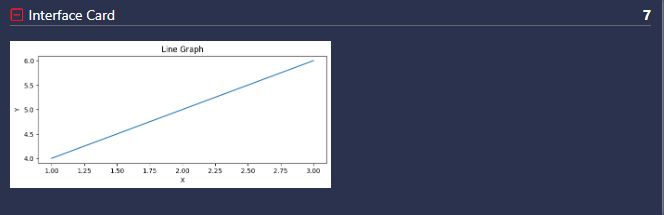# Overview

The line graph output ` @output line-graph` is used to output a variable's value as a line graph. This is typically used with arrays to visualize them in graphical form.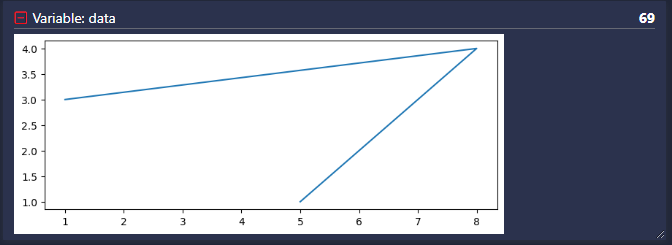# Usage

``````arr = [[1, 3],[8, 4], [5, 1]]
# @output line-graph data=arr
``````
``````x = [1,2,3]
y = [4,5,6]
# @output line-graph data-x=x data-y=y
``````

# Named Arguments

Named Argument

Possible Values

Purpose

xmin

Number

Clips x-axis to start at xmin

xmax

Number

Clips x-axis to end at xmax

ymin

Number

Clips y-axis to start at ymin

ymax

Number

Clips y-axis to end at ymax

title

"Text"

Graph's title

figsize

(width,height)

Defines figure size

xlabel

"Text"

Defines x axis label

ylabel

"Text"

Defines y axis label

size

Custom Pixel Size (eg. "50x100, 50%x*,500x*")

Specifies the percentage width and the height of the output card that it occupies

# Examples

### Displaying Variable As A Line Graph With A 2D Array Input

``````arr = [[1, 3],[8, 4], [5, 1]]
# @output line-graph data=arr
``````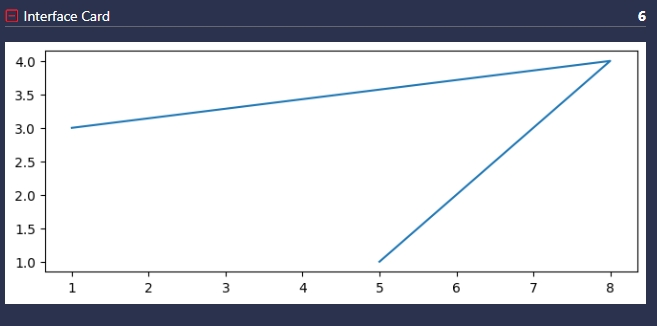### Displaying Variable As A Line Graph With Two 1D Arrays

``````x = [1,2,3]
y = [4,5,6]
# @output line-graph data-x=x data-y=y xlabel="X" ylabel="Y" title="Line Graph" size=100%x*
``````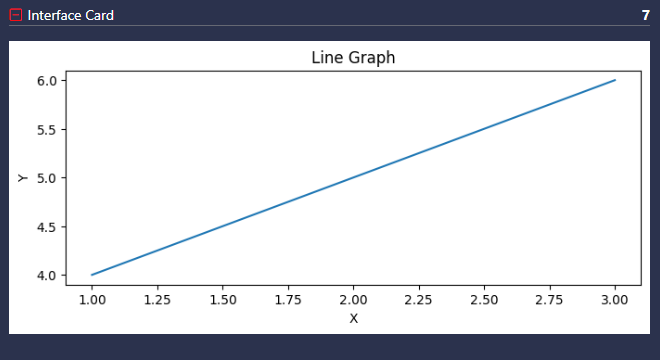### Displaying Variable As A Line Graph - Custom Pixel Size

``````x = [1,2,3]
y = [4,5,6]
# @output line-graph data-x=x data-y=y xlabel="X" ylabel="Y" title="Line Graph" size=300x200
``````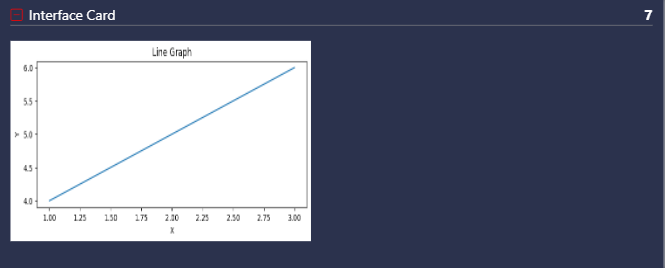### Displaying Variable As A Line Graph - Custom Percentage Size

``````x = [1,2,3]
y = [4,5,6]
# @output line-graph data-x=x data-y=y xlabel="X" ylabel="Y" title="Line Graph" size=50%x*
``````